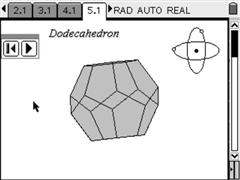PT EN

## Polyhedra 3DIn each page of each of these files you'll find a different polyhedron, drawn in 3D and with the correct perspective. You can rotate them freely or animate the polyhedra.

Due to the large file size, the collection was divided in four parts:

• File 1: cube, tetrahedron, octahedron, dodecahedron and icosahedron.
• File 2: triangular, quadrangular, pentagonal and hexagonal pyramids;
• File 3: triangular, quadrangular, pentagonal and hexagonal prisms and parallelepiped;
• File 4: truncated icosahedron (aka buckyball or football).

Because each step requires a high amount of calculations for the projections, more complex polyhedra will have worse performance than simpler ones. So, polyhedra like the hexagonal prism or the icosahedron (12 vertices), the dodecahedron (20 vertices) or the truncated icosahedron (60 vertices) will perform worse than the tetrahedron or the cube.